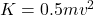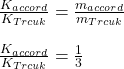Question

accord and a track have the same speed of 30 metre per second if their masses are in the ratio of 1 is to 3 find the ratio of the Kinetic energies ​

1.thucuc

The ratio of kinetic energy of accord to the truck is 1 : 3.

Explanation:

Speed, v = 30 m/s

Ratio of mass of accord to truck = 1 : 3

The kinetic energy is given byAs the speed is same so the ratio of the kinetic energies is same as the ratio of masses.